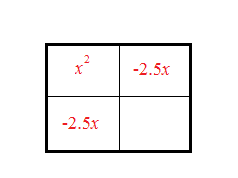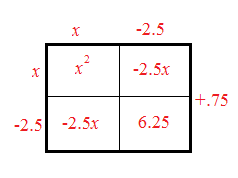### Home > A2C > Chapter 4 > Lesson 4.3.2 > Problem4-149

4-149.

Use the technique of completing the square to express $y = x^{2} − 5x + 7$ in graphing form and state the vertex.

Set up the generic rectangle.

How many ones do you need to complete the square?How many do you have?

Write the factors.